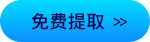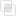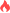python中time模块的时间格式2021-03-06 15:16:33282浏览 · 0收藏 · 0评论1、timestamp时间戳

```#查找此系统的新纪元
print(time.gmtime(0))
#time.struct_time(tm_year=1970, tm_mon=1, tm_mday=1, tm_hour=0, tm_min=0, tm_sec=0, tm_wday=3, tm_yday=1, tm_isdst=0)

#生成当前时间的timestamp
print(time.time())#1556973222.546307```

2、struct_time时间元组

```#生成struct_time
print(time.localtime())#time.struct_time(tm_year=2019, tm_mon=5, tm_mday=11, tm_hour=12, tm_min=20, tm_sec=58, tm_wday=5, tm_yday=131, tm_isdst=0)```

3、format time格式化时间

```#生成format_time
#生成自定义格式的时间表示格式
print(time.strftime("%Y-%m-%d %X",time.localtime()))#2019-05-04 20:40:01
#生成固定格式的时间表示格式
print(time.asctime(time.localtime()))#Sat May 11 19:45:16 2019
print(time.ctime(time.time()))#Sat May 11 19:45:16 2019
print(time.ctime(time.time()+10))#Sat May 11 19:45:26 2019```python中re有哪些常用函数python堆排序是什么?python创建和使用堆的方法python中super的使用注意python如何在自定义类上使用堆排序python模块的搜索路径和顺序python列表的优点探究javascript字符串类型的转换398

PHP使用fread()操作字节431

PHP中define定义常量的方法423